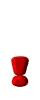Relational Operators

 Operator Description = = equal to ! = not equal to > greater than > = greater than or equal to < less than < = less than or equal to

There are six relational operators used for data comparisons in C++.  These operators must always appear between 2 literals, 2 variables, 2 arithmetic expressions, or a combination of these possibilities.  The operators may be used with numbers, characters, or apstrings.

Check out this example:

 Assume these values are assigned: int a = 4; int b = 12; int c = 16; int d = 4; ... then the following are true: a = = d b < c c > a c != d a >= 0Notice that ONE equal sign is used to "assign" a value, but TWO equal signs are used to check to see if values are equal to one another.

Relational operators always yield a TRUE or FALSE result.
Remember that a TRUE result evaluates to any non-zero value (often 1),
while a FALSE result evaluates only to zero.

Relational operators have a lower precedence than the arithmetic operators.
This means that an expression such as  x + 4 > = y - 5
is the same as (x + 4) > = (y - 5).